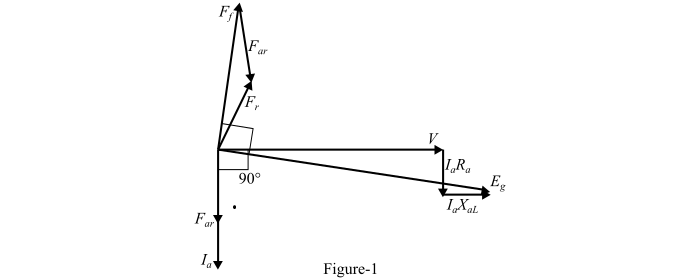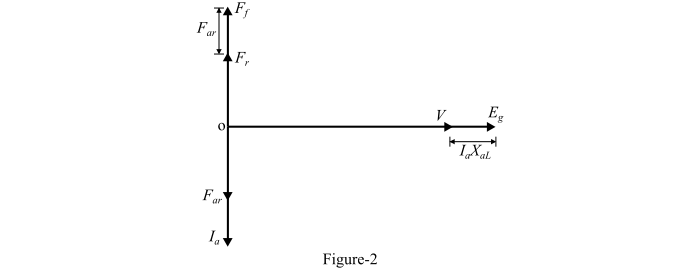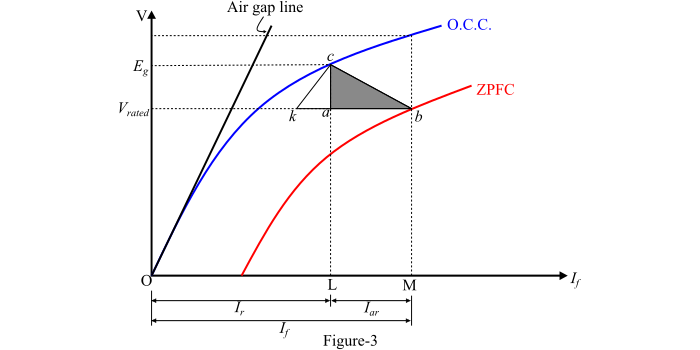# Synchronous Generator ŌĆō Zero Power Factor Characteristics and Potier Triangle

The zero power factor characteristics (ZPFC) is the graph plotted between the armature terminal voltage per phase and the field current, when the machine being operating with constant rated armature current at synchronous speed and zero lagging power factor. The ZPFC is also known as Potier Characteristics after its originator.

In order to maintain the power factor very low, the alternator is loaded by means of reactors or by an under-excited synchronous motor. The shape of the ZPFC is very much like that of the open-circuit characteristic (O.C.C.) displaced downwards and to the right.

## Phasor Diagram

Figure-1 shows the phasor diagram of the alternator corresponding to zero power factor lagging load.Here, the terminal voltage per phase (V) is taken as the reference phasor. At zero power factor lagging, the armature current (­ØÉ╝­ØæÄ) lags behind the voltage V by 90°. The voltage drop (­ØÉ╝­ØæÄ­Øæģ­ØæÄ) in the armature resistance is drawn parallel to the current (­ØÉ╝­ØæÄ) and the drop (­ØÉ╝­ØæÄ­Øæŗ­ØæÄ­ØÉ┐) in the leakage reactance perpendicular to (­ØÉ╝­ØæÄ).

Hence, the generated voltage per phase is then,

$$\mathrm{­ØÉĖ_{­Øæö} = ­Øæē + ­ØÉ╝_{­ØæÄ}­Øæģ_{­ØæÄ} + ­ØÉ╝_{­ØæÄ}­Øæŗ_{­ØæÄL} … (1)}$$

If

• $­ØÉ╣_{­ØæÄ­Øæ¤}$ = Armature reaction MMF (in phase with $­ØÉ╝_{­ØæÄ}$)

• $­ØÉ╣_{­Øæō}$ = Field MMF

• $­ØÉ╣_{­Øæ¤}$ = Resultant MMF in the air gap

The three MMF phasors ­ØÉ╣­Øæō , ­ØÉ╣­Øæ¤ and ­ØÉ╣­ØæÄ­Øæ¤ are in phase and their magnitudes are related by the following equation −

$$\mathrm{­ØÉ╣_{­Øæō} = ­ØÉ╣_{­Øæ¤} + ­ØÉ╣_{­ØæÄ­Øæ¤} … (2)}$$If the armature resistance (­Øæģ­ØæÄ) is neglected, the resulting phasor diagram will be as shown in Figure-2. From Figure-2, it can be seen that the terminal voltage per phase (V), the reactance voltage drop (­ØÉ╝­ØæÄ­Øæŗ­ØæÄ­ØÉ┐) and the generated voltage (­ØÉĖ­Øæö) are all in phase. Hence, the terminal voltage (V) is practically equal to the arithmetical difference between ­ØÉĖ­Øæö and ­ØÉ╝­ØæÄ­Øæŗ­ØæÄ­ØÉ┐i.e.,

$$\mathrm{­Øæē = ­ØÉĖ_{­Øæö} − ­ØÉ╝_{­ØæÄ}­Øæŗ_{­ØæÄ­ØÉ┐} … (3)}$$

The arithmetical expressions given in eqns. (2) and (3) form the basis for the Potier triangle.

Also, Eqn. (2) can be transformed into its equivalent field current form by dividing both sides by the effective number of turns per pole (­Øæć­Øæō) on the rotor.Thus,

$$\mathrm{\frac{­ØÉ╣_{­Øæō}}{­Øæć_{­Øæō}}=\frac{­ØÉ╣_{r}}{­Øæć_{­Øæō}}+\frac{­ØÉ╣_{­ØæÄ­Øæ¤}}{­Øæć_{­Øæō}}}$$

$$\mathrm{\Rightarrow\:­ØÉ╝_{­Øæō} = ­ØÉ╝_{­Øæ¤} + ­ØÉ╝_{­ØæÄ­Øæ¤} … (4)}$$

## Potier Triangle

The O.C.C. and ZPFC are shown in Figure-3.Consider a point b on the ZPFC corresponding to the rated terminal voltage (V) and a field current of (­Øæé­ØæĆ = ­ØÉ╝­Øæō).

If under this operating condition, the armature reaction MMF (­ØÉ╣­ØæÄ­Øæ¤) has a value expressed in equivalent field current of (­ØÉ┐­ØæĆ = ­ØÉ╝­ØæÄ­Øæ¤), then the equivalent field current of the resultant MMF (­ØÉ╣­Øæ¤) would be (­Øæé­ØÉ┐ = ­ØÉ╝­Øæ¤).

This resultant field current (­Øæé­ØÉ┐ = ­ØÉ╝­Øæ¤) would result in a generated voltage (­ØÉ┐­ØæÉ = ­ØÉĖ­Øæö) from the O.C.C. Since, for zero power factor lagging operation, the generated voltage is given by,

$$\mathrm{­Øæ¼_{­Øæö} = ­ØæĮ + ­Øæ░_{­ØæÄ}­Øæ┐_{­ØæÄ­ØÉ┐} … (5)}$$

Here, the vertical distance ac must be equal to the leakage reactance voltage drop (­ØÉ╝­ØæÄ­Øæŗ­ØæÄ­ØÉ┐) where ­ØÉ╝­ØæÄ is the rated armature current. Therefore, the armature leakage reactance is,

$$\mathrm{­Øæŗ_{­ØæÄ­ØÉ┐} =\frac{Voltage\:­ØæÄ­ØæÉ\:per \:phase}{Rated\:armature\:current}… (6)}$$

Now, the triangle formed by the vertices a, b and c is known as the Potier Triangle.

In the synchronous machine, the effect of the field leakage flux in combination with the armature leakage flux gives rise to an equivalent leakage reactance ­Øæŗ­ØæØ, known as Potier Reactance. It is given by,

$$\mathrm{­Øæŗ_{­ØæØ} =\frac{Voltage\:drop\:per\:phase (voltage\:­ØæÄ­ØæÉ)}{ZPF\:rated\:armature\:current\:per\: phase (­ØÉ╝_{­ØæÄ})}… (7)}$$

For a cylindrical rotor synchronous machine, the Potier reactance (­Øæŗ­ØæØ) is approximately equal to the armature leakage reactance (­Øæŗ­ØæÄ­ØÉ┐) while in a salient-pole machine, the ­Øæŗ­ØæØ may be as large as 3 times ­Øæŗ­ØæÄ­ØÉ┐.